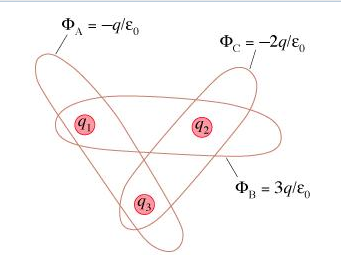# Problem: The figure shows three Gaussian surfaces and the electric flux through each.A. What is the charge q1?B. What is the charge q2?C. What is the charge q3?

###### FREE Expert Solution
85% (328 ratings)###### Problem Details

The figure shows three Gaussian surfaces and the electric flux through each.A. What is the charge q1?

B. What is the charge q2?

C. What is the charge q3?